# 从FM推演各深度CTR预估模型(附代码)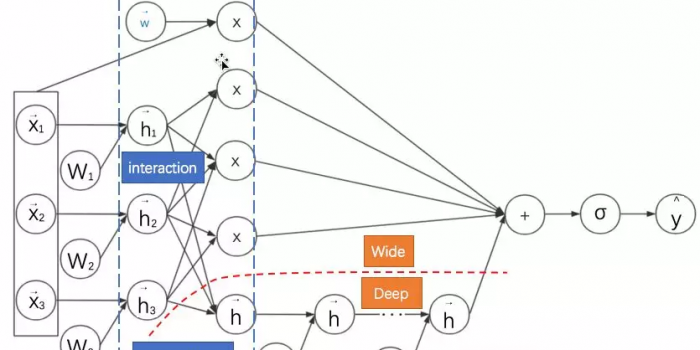1.引言

2.本文的思路与方法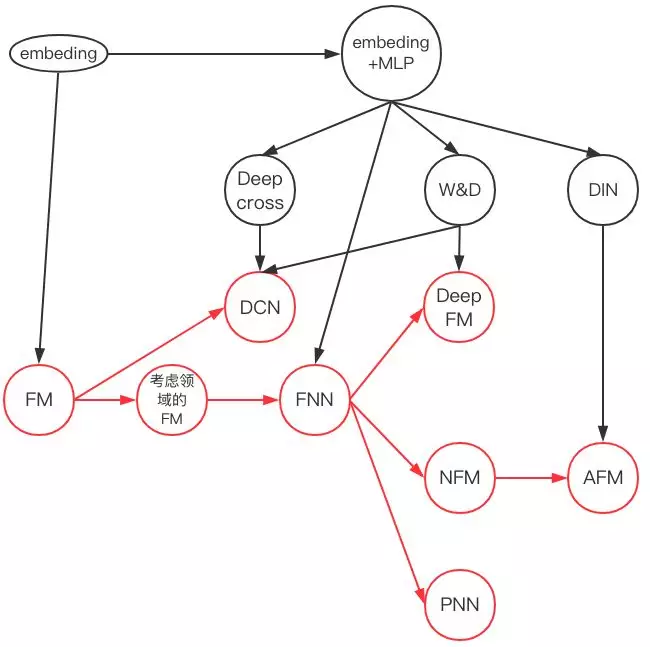3.FM：降维版本的特征二阶组合

CTR预估本质是一个二分类问题，以移动端展示广告推荐为例，依据日志中的用户侧的信息（比如年龄，性别，国籍，手机上安装的app列表）、广告侧的信息（广告id，广告类别，广告标题等）、上下文侧信息（渠道id等），去建模预测用户是否会点击该广告。
FM出现之前的传统的处理方法是人工特征工程加上线性模型（如逻辑回归Logistic Regression）。为了提高模型效果，关键技术是找到到用户点击行为背后隐含的特征组合。如男性、大学生用户往往会点击游戏类广告，因此“男性且是大学生且是游戏类”的特征组合就是一个关键特征。但这本质仍是线性模型，其假设函数表示成内积形式一般为：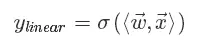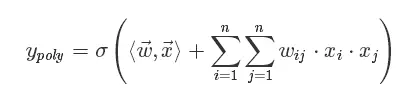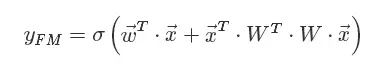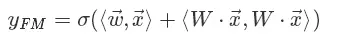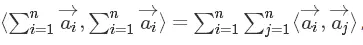https://pan.baidu.com/s/1eDwOxweRDPurI2fF51EALQ``` class FM(Model):

def __init__(self, input_dim=None, output_dim=1, factor_order=10, init_path=None, opt_algo='gd', learning_rate=1e-2,

l2_w=0, l2_v=0, random_seed=None):

Model.__init__(self)

# 一次、二次交叉、偏置项

init_vars = [('w', [input_dim, output_dim], 'xavier', dtype),

('v', [input_dim, factor_order], 'xavier', dtype),

('b', [output_dim], 'zero', dtype)]

self.graph = tf.Graph()

with self.graph.as_default():

if random_seed is not None:

tf.set_random_seed(random_seed)

self.X = tf.sparse_placeholder(dtype)

self.y = tf.placeholder(dtype)

self.vars = init_var_map(init_vars, init_path)

w = self.vars['w']

v = self.vars['v']

b = self.vars['b']

# [(x1+x2+x3)^2 - (x1^2+x2^2+x3^2)]/2

# 先计算所有的交叉项，再减去平方项(自己和自己相乘)

X_square = tf.SparseTensor(self.X.indices, tf.square(self.X.values), tf.to_int64(tf.shape(self.X)))

xv = tf.square(tf.sparse_tensor_dense_matmul(self.X, v))

p = 0.5 * tf.reshape(

tf.reduce_sum(xv - tf.sparse_tensor_dense_matmul(X_square, tf.square(v)), 1),

[-1, output_dim])

xw = tf.sparse_tensor_dense_matmul(self.X, w)

logits = tf.reshape(xw + b + p, [-1])

self.y_prob = tf.sigmoid(logits)

self.loss = tf.reduce_mean(

tf.nn.sigmoid_cross_entropy_with_logits(logits=logits, labels=self.y)) + \

l2_w * tf.nn.l2_loss(xw) + \

l2_v * tf.nn.l2_loss(xv)

self.optimizer = get_optimizer(opt_algo, learning_rate, self.loss)

#GPU设定

config = tf.ConfigProto()

config.gpu_options.allow_growth = True

self.sess = tf.Session(config=config)

# 图中所有variable初始化

tf.global_variables_initializer().run(session=self.sess) ```

4.用神经网络的视角看FM：嵌入后再进行内积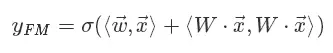• FM首先是对离散特征进行嵌入。
• 之后通过对嵌入后的稠密向量进行内积来进行二阶特征组合。
• 最后再与线性模型的结果求和进而得到预估点击率。
其示意图如下。为了表述清晰，我们绘制的是神经网络计算图而不是网络结构图——在网络结构图中增加了权重WW的位置。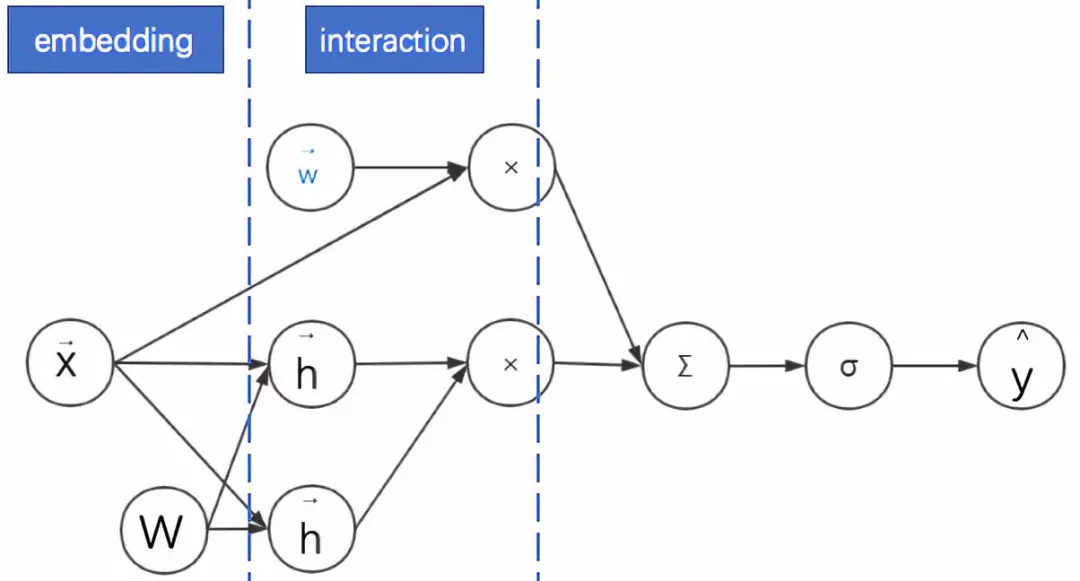5.FM的实际应用：考虑领域信息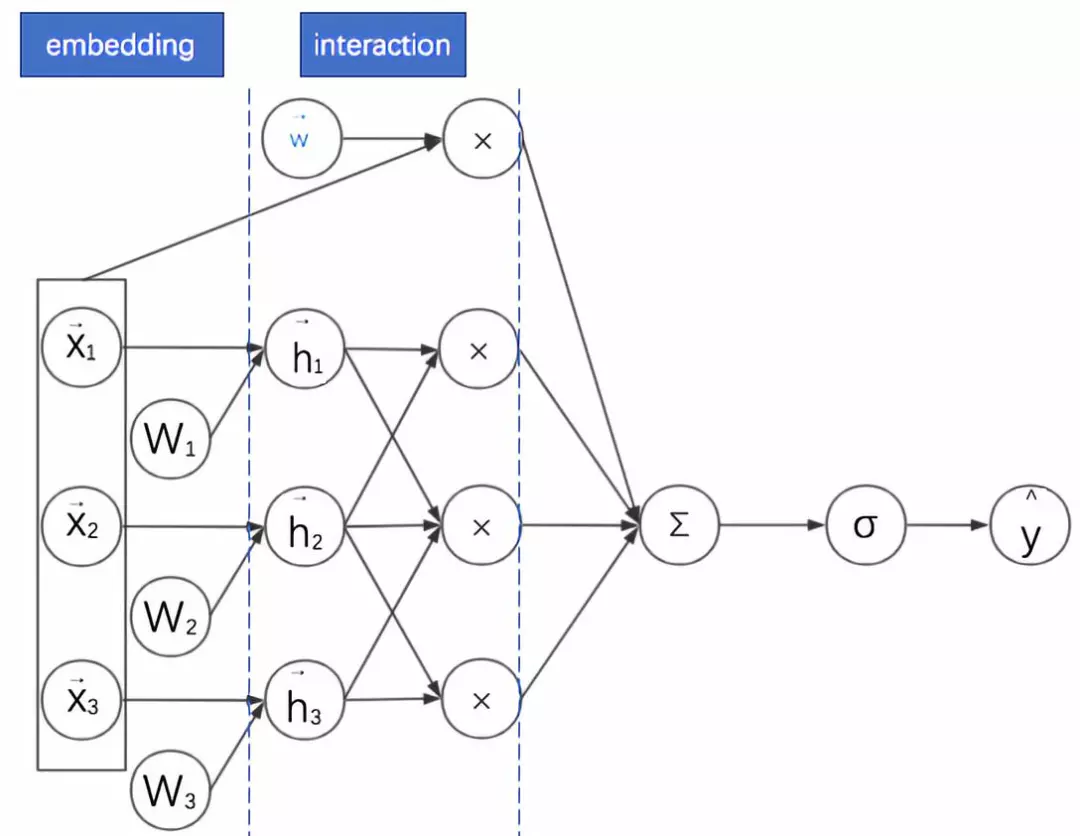6.embedding+MLP：深度学习CTR预估的通用框架

embedding+MLP是对于分领域离散特征进行深度学习CTR预估的通用框架。深度学习在特征组合挖掘(特征学习)方面具有很大的优势。比如以CNN为代表的深度网络主要用于图像、语音等稠密特征上的学习，以W2V、RNN为代表的深度网络主要用于文本的同质化、序列化高维稀疏特征的学习。CTR预估的主要场景是对离散且有具体领域的特征进行学习，所以其深度网络结构也不同于CNN与RNN。

• 对不同领域的one-hot特征进行嵌入（embedding），使其降维成低维度稠密特征。
• 然后将这些特征向量拼接（concatenate）成一个隐含层。
• 之后再不断堆叠全连接层，也就是多层感知机(Multilayer Perceptron, MLP，有时也叫作前馈神经网络)。
• 最终输出预测的点击率。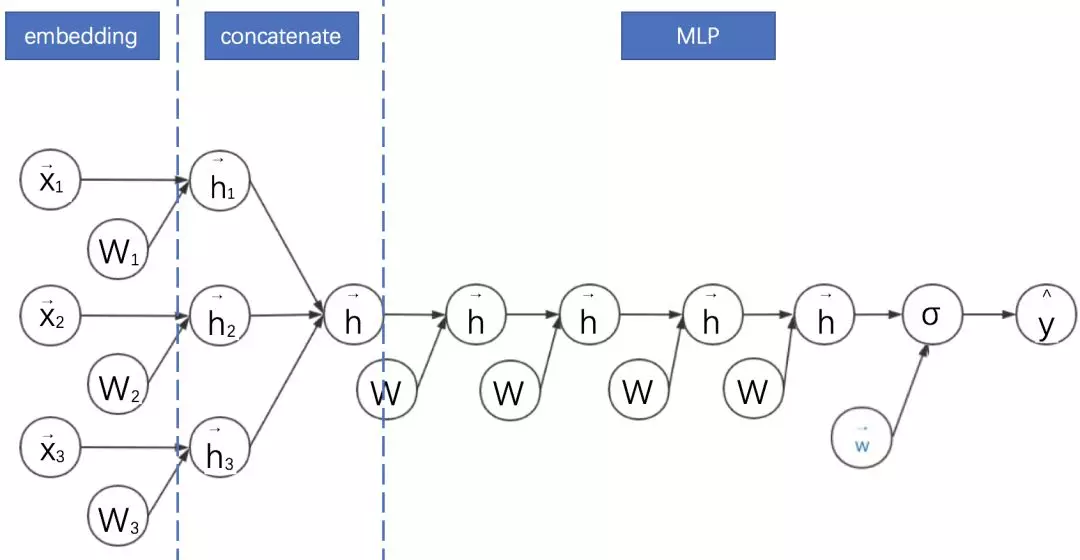embedding+MLP的缺点是只学习高阶特征组合，对于低阶或者手动的特征组合不够兼容，而且参数较多，学习较困难。

7.FNN:FM与MLP的串联结合

Weinan Zhang等在2016年提出的因子分解机神经网络(Factorisation Machine supported Neural Network，FNN)将考FM与MLP进行了结合。它有着十分显著的特点：

• 采用FM预训练得到的隐含层及其权重作为神经网络的第一层的初始值，之后再不断堆叠全连接层，最终输出预测的点击率。
• 可以将FNN理解成一种特殊的embedding+MLP，其要求第一层嵌入后的各领域特征维度一致，并且嵌入权重的初始化是FM预训练好的。
• 这不是一个端到端的训练过程，有贪心训练的思路。而且如果不考虑预训练过程，模型网络结构也没有考虑低阶特征组合。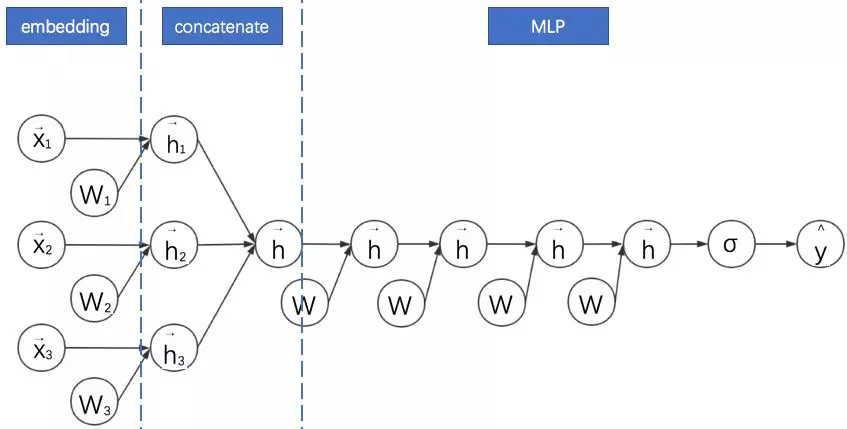https://pan.baidu.com/s/1eDwOxweRDPurI2fF51EALQ

``` class FNN(Model):

def __init__(self, field_sizes=None, embed_size=10, layer_sizes=None, layer_acts=None, drop_out=None,

embed_l2=None, layer_l2=None, init_path=None, opt_algo='gd', learning_rate=1e-2, random_seed=None):

Model.__init__(self)

init_vars = []

num_inputs = len(field_sizes)

for i in range(num_inputs):

init_vars.append(('embed_%d' % i, [field_sizes[i], embed_size], 'xavier', dtype))

node_in = num_inputs * embed_size

for i in range(len(layer_sizes)):

init_vars.append(('w%d' % i, [node_in, layer_sizes[i]], 'xavier', dtype))

init_vars.append(('b%d' % i, [layer_sizes[i]], 'zero', dtype))

node_in = layer_sizes[i]

self.graph = tf.Graph()

with self.graph.as_default():

if random_seed is not None:

tf.set_random_seed(random_seed)

self.X = [tf.sparse_placeholder(dtype) for i in range(num_inputs)]

self.y = tf.placeholder(dtype)

self.keep_prob_train = 1 - np.array(drop_out)

self.keep_prob_test = np.ones_like(drop_out)

self.layer_keeps = tf.placeholder(dtype)

self.vars = init_var_map(init_vars, init_path)

w0 = [self.vars['embed_%d' % i] for i in range(num_inputs)]

xw = tf.concat([tf.sparse_tensor_dense_matmul(self.X[i], w0[i]) for i in range(num_inputs)], 1)

l = xw

#全连接部分

for i in range(len(layer_sizes)):

wi = self.vars['w%d' % i]

bi = self.vars['b%d' % i]

print(l.shape, wi.shape, bi.shape)

l = tf.nn.dropout(

activate(

tf.matmul(l, wi) + bi,

layer_acts[i]),

self.layer_keeps[i])

l = tf.squeeze(l)

self.y_prob = tf.sigmoid(l)

self.loss = tf.reduce_mean(

tf.nn.sigmoid_cross_entropy_with_logits(logits=l, labels=self.y))

if layer_l2 is not None:

self.loss += embed_l2 * tf.nn.l2_loss(xw)

for i in range(len(layer_sizes)):

wi = self.vars['w%d' % i]

self.loss += layer_l2[i] * tf.nn.l2_loss(wi)

self.optimizer = get_optimizer(opt_algo, learning_rate, self.loss)

config = tf.ConfigProto()

config.gpu_options.allow_growth = True

self.sess = tf.Session(config=config)

tf.global_variables_initializer().run(session=self.sess) ```

8.DeepFM: FM与MLP的并联结合

• 不需要预训练。
• 将考虑领域信息的FM部分与MLP部分并联起来（借用初中电路的术语），其实就是对两个模型进行联合训练。
• 考虑领域信息的FM部分的嵌入向量拼接起来作为MLP部分的输入特征，也就是是两个模型共享嵌入后的特征。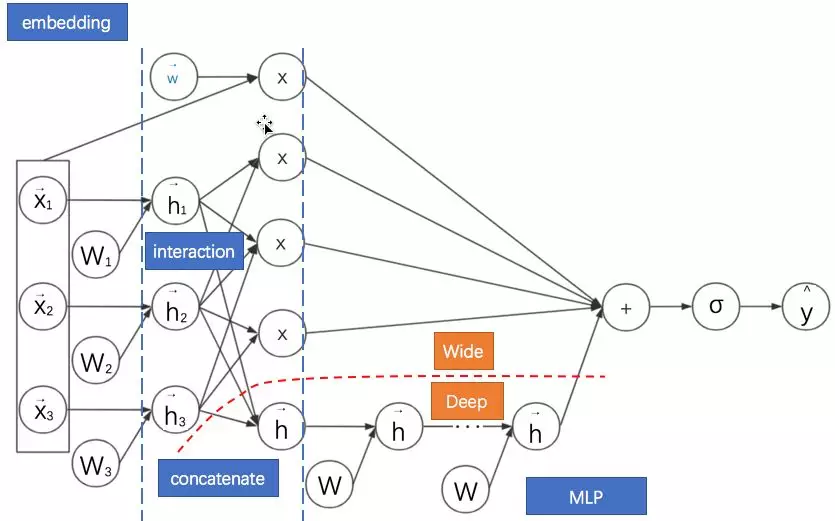9.NFM:通过逐元素乘法延迟FM的实现过程NFM的基本特点是：

• 利用二阶交互池化层（Bi-Interaction Pooling）对FM嵌入后的向量两两进行元素级别的乘法，形成同维度的向量求和后作为前馈神经网络的输入。计算图中用圈乘⨂表示逐元素乘法运算。
• NFM与DeepFM的区别是没有单独的FM的浅层网络进行联合训练，而是将其整合后直接输出给前馈神经网络。
• 当MLP的全连接层都是恒等变换且最后一层参数全为1时，NFM就退化成了FM。可见，NFM是FM的推广，它推迟了FM的实现过程，并在其中加入了更多非线性运算。
• 另一方面，我们观察计算图会发现NFM与FNN非常相似。它们的主要区别是NFM在embedding之后对特征进行了两两逐元素乘法。因为逐元素相乘的向量维数不变，之后对这些向量求和的维数仍然与embedding的维数一致。因此输入到MLP的参数比起直接concatenate的FNN更少。

https://pan.baidu.com/s/1eDwOxweRDPurI2fF51EALQ

``` def model_fn(features, labels, mode, params):

"""Bulid Model function f(x) for Estimator."""

#------hyperparameters----

field_size = params["field_size"]

feature_size = params["feature_size"]

embedding_size = params["embedding_size"]

l2_reg = params["l2_reg"]

learning_rate = params["learning_rate"]

#optimizer = params["optimizer"]

layers = map(int, params["deep_layers"].split(','))

dropout = map(float, params["dropout"].split(','))

#------bulid weights------

Global_Bias = tf.get_variable(name='bias', shape=, initializer=tf.constant_initializer(0.0))

Feat_Bias = tf.get_variable(name='linear', shape=[feature_size], initializer=tf.glorot_normal_initializer())

Feat_Emb = tf.get_variable(name='emb', shape=[feature_size,embedding_size], initializer=tf.glorot_normal_initializer())

#------build feaure-------

feat_ids  = features['feat_ids']

feat_ids = tf.reshape(feat_ids,shape=[-1,field_size])

feat_vals = features['feat_vals']

feat_vals = tf.reshape(feat_vals,shape=[-1,field_size])

#------build f(x)------

with tf.variable_scope("Linear-part"):

feat_wgts = tf.nn.embedding_lookup(Feat_Bias, feat_ids)         # None * F * 1

y_linear = tf.reduce_sum(tf.multiply(feat_wgts, feat_vals),1)

with tf.variable_scope("BiInter-part"):

embeddings = tf.nn.embedding_lookup(Feat_Emb, feat_ids)         # None * F * K

feat_vals = tf.reshape(feat_vals, shape=[-1, field_size, 1])

embeddings = tf.multiply(embeddings, feat_vals)                 # vij * xi

sum_square_emb = tf.square(tf.reduce_sum(embeddings,1))

square_sum_emb = tf.reduce_sum(tf.square(embeddings),1)

deep_inputs = 0.5*tf.subtract(sum_square_emb, square_sum_emb)   # None * K

with tf.variable_scope("Deep-part"):

if mode == tf.estimator.ModeKeys.TRAIN:

train_phase = True

else:

train_phase = False

if mode == tf.estimator.ModeKeys.TRAIN:

deep_inputs = tf.nn.dropout(deep_inputs, keep_prob=dropout)                      # None * K

for i in range(len(layers)):

deep_inputs = tf.contrib.layers.fully_connected(inputs=deep_inputs, num_outputs=layers[i], \

weights_regularizer=tf.contrib.layers.l2_regularizer(l2_reg), scope='mlp%d' % i)

if FLAGS.batch_norm:

deep_inputs = batch_norm_layer(deep_inputs, train_phase=train_phase, scope_bn='bn_%d' %i)   #放在RELU之后 https://github.com/ducha-aiki/caffenet-benchmark/blob/master/batchnorm.md#bn----before-or-after-relu

if mode == tf.estimator.ModeKeys.TRAIN:

deep_inputs = tf.nn.dropout(deep_inputs, keep_prob=dropout[i])                              #Apply Dropout after all BN layers and set dropout=0.8(drop_ratio=0.2)

#deep_inputs = tf.layers.dropout(inputs=deep_inputs, rate=dropout[i], training=mode == tf.estimator.ModeKeys.TRAIN)

y_deep = tf.contrib.layers.fully_connected(inputs=deep_inputs, num_outputs=1, activation_fn=tf.identity, \

weights_regularizer=tf.contrib.layers.l2_regularizer(l2_reg), scope='deep_out')

y_d = tf.reshape(y_deep,shape=[-1])

with tf.variable_scope("NFM-out"):

#y_bias = Global_Bias * tf.ones_like(labels, dtype=tf.float32)  # None * 1  warning;这里不能用label，否则调用predict/export函数会出错，train/evaluate正常；初步判断estimator做了优化，用不到label时不传

y_bias = Global_Bias * tf.ones_like(y_d, dtype=tf.float32)      # None * 1

y = y_bias + y_linear + y_d

pred = tf.sigmoid(y)

predictions={"prob": pred}

export_outputs = {tf.saved_model.signature_constants.DEFAULT_SERVING_SIGNATURE_DEF_KEY: tf.estimator.export.PredictOutput(predictions)}

# Provide an estimator spec for `ModeKeys.PREDICT`

if mode == tf.estimator.ModeKeys.PREDICT:

return tf.estimator.EstimatorSpec(

mode=mode,

predictions=predictions,

export_outputs=export_outputs)

#------bulid loss------

loss = tf.reduce_mean(tf.nn.sigmoid_cross_entropy_with_logits(logits=y, labels=labels)) + \

l2_reg * tf.nn.l2_loss(Feat_Bias) + l2_reg * tf.nn.l2_loss(Feat_Emb)

# Provide an estimator spec for `ModeKeys.EVAL`

eval_metric_ops = {

"auc": tf.metrics.auc(labels, pred)

}

if mode == tf.estimator.ModeKeys.EVAL:

return tf.estimator.EstimatorSpec(

mode=mode,

predictions=predictions,

loss=loss,

eval_metric_ops=eval_metric_ops)

#------bulid optimizer------

optimizer = tf.train.AdamOptimizer(learning_rate=learning_rate, beta1=0.9, beta2=0.999, epsilon=1e-8)

elif FLAGS.optimizer == 'Momentum':

optimizer = tf.train.MomentumOptimizer(learning_rate=learning_rate, momentum=0.95)

elif FLAGS.optimizer == 'ftrl':

optimizer = tf.train.FtrlOptimizer(learning_rate)

train_op = optimizer.minimize(loss, global_step=tf.train.get_global_step())

# Provide an estimator spec for `ModeKeys.TRAIN` modes

if mode == tf.estimator.ModeKeys.TRAIN:

return tf.estimator.EstimatorSpec(

mode=mode,

predictions=predictions,

loss=loss,

train_op=train_op)  ```

10.AFM: 对简化版NFM进行加权求和

NFM的主要创新点是在FM过程中添加了逐元素相乘的运算来增加模型的复杂度。但没有在此基础上添加更复杂的运算过程，比如对加权求和。Jun Xiao等在2017年提出了注意力因子分解模型（Attentional Factorization Machine，AFM）就是在这个方向上的改进。其计算图如下所示：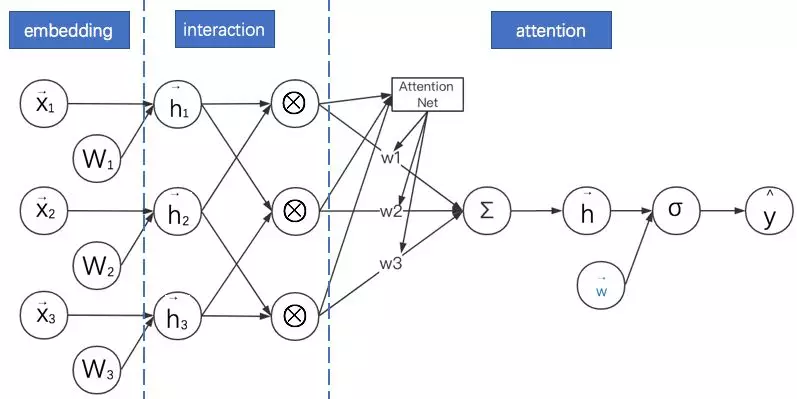AFM的特点是：

• AFM与NFM都是致力于充分利用二阶特征组合的信息，对嵌入后的向量两两进行逐元素乘法，形成同维度的向量。而且AFM没有MLP部分。
• AFM通过在逐元素乘法之后形成的向量进行加权求和，而且权重是基于网络自身来产生的。其方法是引入一个注意力子网络（Attention Net）。
• 当权重都相等时，AFM退化成无全连接层的NFM。
• “注意力子网络”的主要操作是进行矩阵乘法，其最终输出结果为softmax，以保证各分量的权重本身是一个概率分布。

11.PNN:通过改进向量乘法运算延迟FM的实现过程• 利用二阶向量积层（Pair-wisely Connected Product Layer）对FM嵌入后的向量两两进行向量积，形成的结果作为之后MLP的输入。计算图中用圆点•表示向量积运算。PNN采用的向量积有内积与外积两种形式。
• 需要说明的是，本计算图中省略了PNN中向量与常数1进行的乘法运算。这部分其实与FNN类似，不是PNN的主要创新点。故在此图中省略。
• 对于内积形式的PNN，因为两个向量相乘的结果为标量，可以直接把各个标量“拼接”成一个大向量，就可以作为MLP的输入了。
• 当MLP的全连接层都是恒等变换且最后一层参数全为1时，内积形式的PNN就退化成了FM。
• 对于外积形式的PNN，因为两个向量相乘相当于列向量与行向量进行矩阵相乘，得到的结果为一个矩阵。各个矩阵向之前内积形式的操作一样直接拼接起来维数太多，论文的简化方案是直接对各个矩阵进行求和，得到的新矩阵（可以理解成之后对其拉长成向量）就直接作为MLP的输入。
• 观察计算图发现外积形式的PNN与NFM很像，其实就是PNN把NFM的逐元素乘法换成了外积。

https://pan.baidu.com/s/1eDwOxweRDPurI2fF51EALQ

12.DCN:高阶FM的降维实现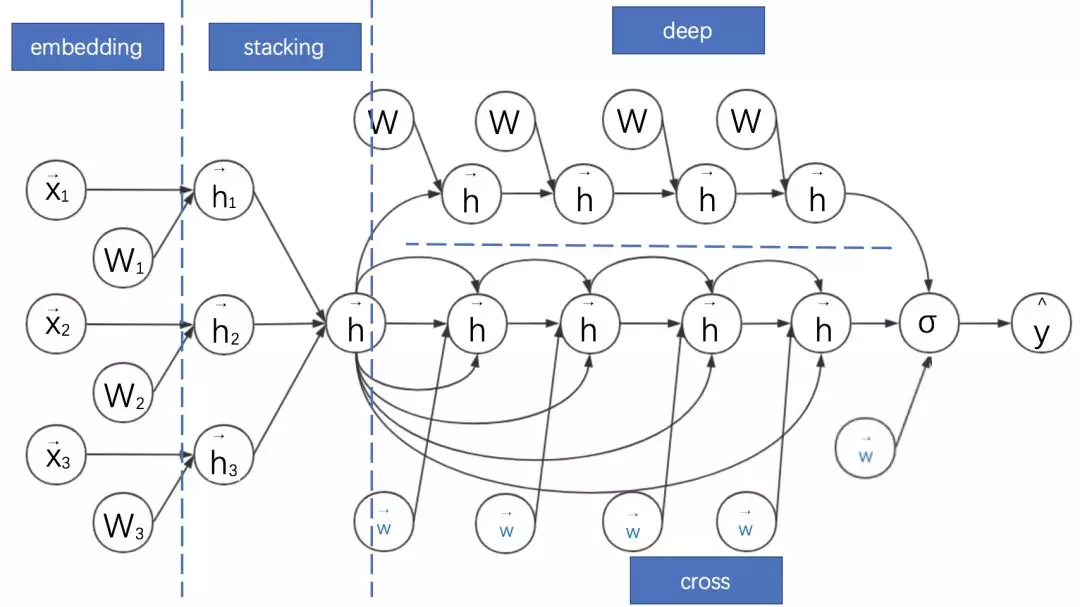DCN的特点如下：

• Deep部分就是普通的MLP网络，主要是全连接。
• 与DeepFM类似，DCN是由embedding+MLP部分与cross部分进行联合训练的。Cross部分是对FM部分的推广。
• cross部分的公式如下：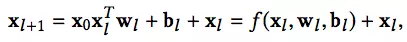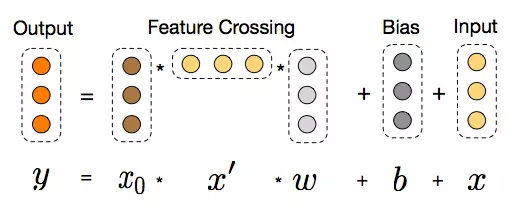• 可以证明，cross网络是FM的过程在高阶特征组合的推广。完全的证明需要一些公式推导，感兴趣的同学可以直接参考原论文的附录。
1. 而用简单的公式证明可以得到一个很重要的结论：只有两层且第一层与最后一层权重参数相等时的Cross网络与简化版FM等价。
• 此处对应简化版的FM视角是将拼接好的稠密向量作为输入向量，且不做领域方面的区分（但产生这些稠密向量的过程是考虑领域信息的，相对全特征维度的全连接层减少了大量参数，可以视作稀疏链接思想的体现）。而且之后进行embedding权重矩阵W只有一列——是退化成列向量的情形。
• 与MLP网络相比，Cross部分在增加高阶特征组合的同时减少了参数的个数，并省去了非线性激活函数。

13.Wide&Deep: DeepFM与DCN的基础框架

Wide&Deep将深度模型与线性模型进行联合训练，二者的结果求和输出为最终点击率。其计算图如下：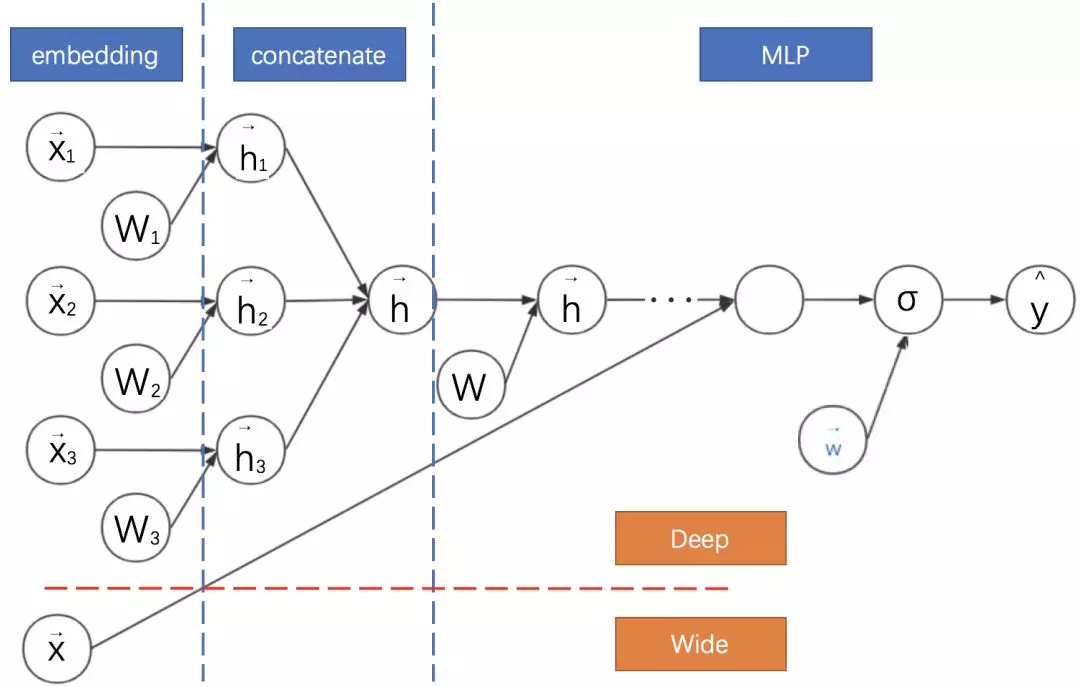• Wide&Deep是前面介绍模型DeepFM与DCN的基础框架。这些模型均采用神经网络联合训练的思路，对神经网络进行并联。
• DeepFM、DCN与Wide&Deep的Deep部分都是MLP。
• Wide&Deep的Wide部分是逻辑回归，可以手动设计组合特征。
• DeepFM的Wide部分是FM，DCN的Wide部分是Cross网络，二者均不强求手动设计特征。但此时都与字面意义上的Wide有一定差异，因为均共享了降维后的嵌入特征。

https://pan.baidu.com/s/1eDwOxweRDPurI2fF51EALQ

``` def get_model(model_type, model_dir):

print("Model directory = %s" % model_dir)

# 对checkpoint去做设定

runconfig = tf.contrib.learn.RunConfig(

save_checkpoints_secs=None,

save_checkpoints_steps = 100,

)

m = None

# 宽模型

if model_type == 'WIDE':

m = tf.contrib.learn.LinearClassifier(

model_dir=model_dir,

feature_columns=wide_columns)

# 深度模型

if model_type == 'DEEP':

m = tf.contrib.learn.DNNClassifier(

model_dir=model_dir,

feature_columns=deep_columns,

hidden_units=[100, 50, 25])

# 宽度深度模型

if model_type == 'WIDE_AND_DEEP':

m = tf.contrib.learn.DNNLinearCombinedClassifier(

model_dir=model_dir,

linear_feature_columns=wide_columns,

dnn_feature_columns=deep_columns,

dnn_hidden_units=[100, 70, 50, 25],

config=runconfig)

print('estimator built')

return m  ```

14.Deep Cross: DCN由其残差网络思想进化

Deep Cross的计算图如下：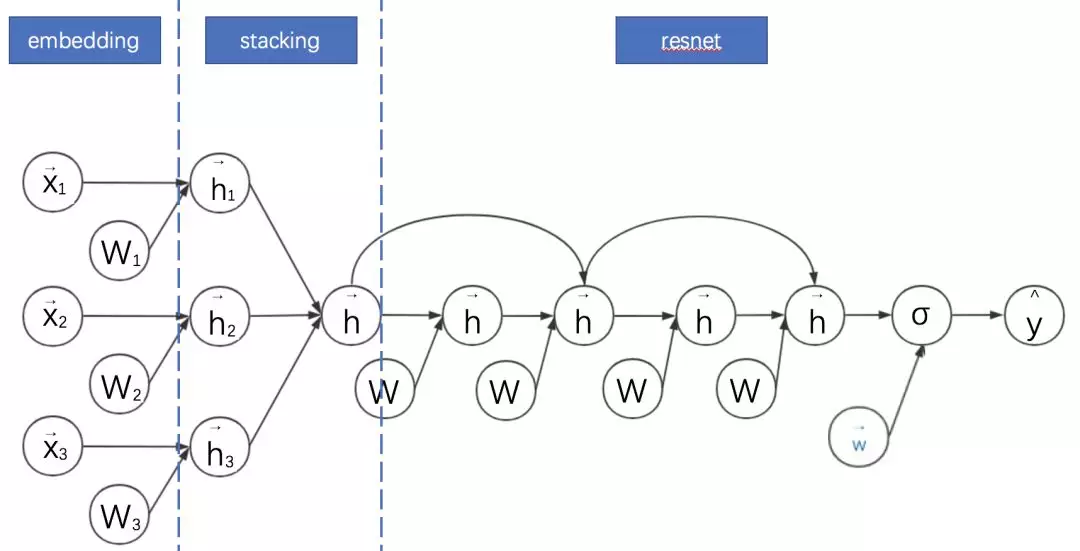• 对embedding+MLP的改进主要是MLP部分增加跳跃连接成为残差网络。
• Deep Cross 与传统的残差网络的区别主要是没有采用卷积操作。其中一个原因是在广告点击率预估领域，特征不具备平移不变性。
• DCN其实是从Deep Cross进化出来的版本。DCN相对Deep Cross的主要贡献是解耦了Deep 与Cross（特征交叉）部分。
• 因此DCN中的Cross部分可以理解为残差网络的变体：其将Deep Cross的跨越链接缩短为只有一层，而全连接部分改为与权重向量和输入向量的内积。

15.DIN:对同领域历史信息引入注意力机制的MLP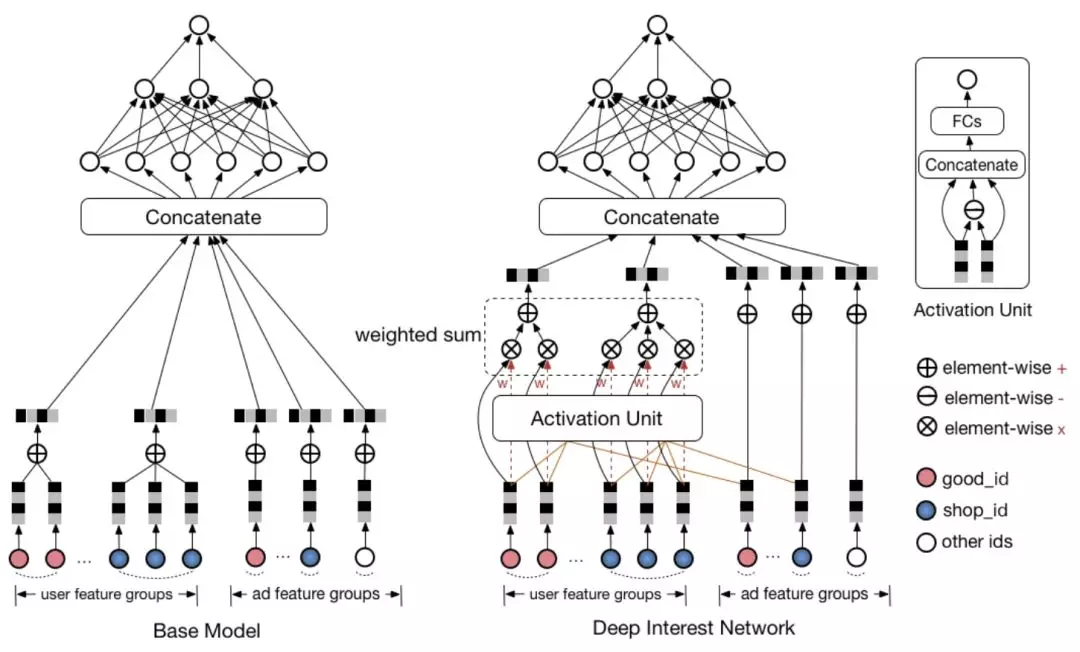• 此处采用原论文的结构图，表示起来更清晰。
• DIN考虑对同一领域的历史特征进行加权求和，以加强其感兴趣的特征的影响。
• 用户的每个领域的历史特征权重则由该历史特征及其对应备选广告特征通过一个子网络得到。即用户历史浏览的商户特征与当前浏览商户特征对应，历史浏览的商品特征与当前浏览商品特征对应。
• 权重子网络主要包括特征之间的元素级别的乘法、加法和全连接等操作。
• AFM也引入了注意力机制。但是AFM是将注意力机制与FM同领域特征求和之后进行结合，DIN直接是将注意力机制与同领域特征求和之前进行结合。

16.多任务视角：信息的迁移与补充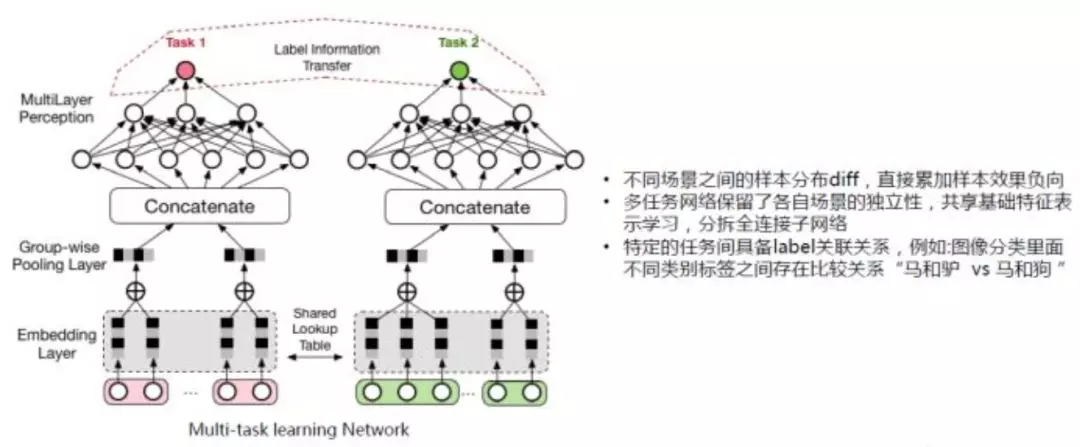DeepFM和DCN也用到了这个思路！只是它们是对同一任务的不同模型进行结合，而多任务学习是对不同任务的不同模型进行结合。而且，我们可以玩得更加复杂。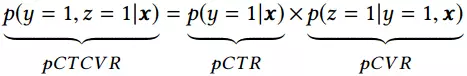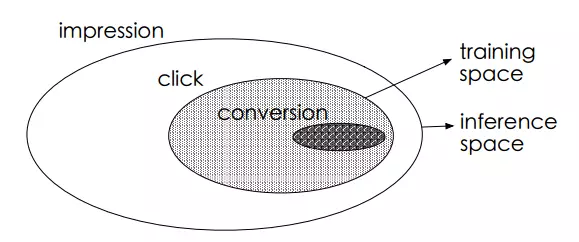ESMM模型提出了下述的网络结构进行问题建模EMMS的特点是：

• 在整个样本空间建模。pCVR 可以在先估计出pCTR 和pCTCVR之后计算得出，如下述公式。从原理上看，相当于分别单独训练两个模型拟合出pCTR 和pCTCVR，进而计算得到pCVR 。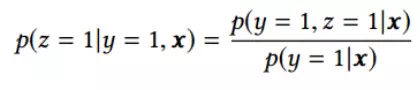17.各种模型的对比和总结• FM其实是对嵌入特征进行两两内积实现特征二阶组合；FNN在FM基础上引入了MLP；
• DeepFM通过联合训练、嵌入特征共享来兼顾FM部分与MLP部分不同的特征组合机制；
• NFM、PNN则是通过改造向量积的方式来延迟FM的实现过程，在其中添加非线性成分来提升模型表现力；
• AFM更进一步，直接通过子网络来对嵌入向量的两两逐元素乘积进行加权求和，以实现不同组合的差异化，也是一种延迟FM实现的方式；
• DCN则是将FM进行高阶特征组合的方向上进行推广，并结合MLP的全连接式的高阶特征组合机制；
• Wide&Deep是兼容手工特征组合与MLP的特征组合方式，是许多模型的基础框架；
• Deep Cross是引入残差网络机制的前馈神经网络，给高维的MLP特征组合增加了低维的特征组合形式，启发了DCN；
• DIN则是对用户侧的某历史特征和广告侧的同领域特征进行组合，组合成的权重反过来重新影响用户侧的该领域各历史特征的求和过程；
• 多任务视角则是更加宏观的思路，结合不同任务（而不仅是同任务的不同模型）对特征的组合过程，以提高模型的泛化能力。

18.后记

• 陈巧红,余仕敏,贾宇波. 广告点击率预估技术综述[J]. 浙江理工大学学报. 2015(11).
• 纪文迪,王晓玲,周傲英. 广告点击率估算技术综述[J]. 华东师范大学学报(自然科学版). 2013(03).
• Rendle S. Factorization machines. Data Mining (ICDM), 2010 IEEE 10th International Conference on. 2010.
• Heng-Tze Cheng and Levent Koc. Wide & deep learning for recommender systems. In Proceedings of the 1st Workshop on Deep Learning for Recommender Systems, pages 7–10. ACM, 2016.
• Weinan Zhang, Tianming Du, and Jun Wang. Deep learning over multi-field categorical data - - A case study on user response prediction. In ECIR, 2016.
• Huifeng Guo, Ruiming Tang, Yunming Ye, Zhenguo Li, and Xiuqiang He. DeepFM: A Factorization-Machine based Neural Network for CTR Prediction. arXiv preprint arXiv:1703.04247 (2017).
• Xiangnan He and Tat-Seng Chua. Neural Factorization Machines for Sparse Predictive Analytics SIGIR. 355–364. 2017.
• Guorui Zhou, Chengru Song, Xiaoqiang Zhu, Xiao Ma, Yanghui Yan, Xingya Dai, Han Zhu, Junqi Jin, Han Li, and Kun Gai. 2017. Deep Interest Network for Click-Through Rate Prediction. arXiv preprint arXiv:1706.06978 (2017).
• J. Xiao, H. Ye, X. He, H. Zhang, F. Wu, and T.-S. Chua. Attentional factorization machines: Learning the weight of feature interactions via attention networks. In IJCAI, 2017.
• Ying Shan, T Ryan Hoens, Jian Jiao, Haijing Wang, Dong Yu, and JC Mao. 2016. Deep Crossing: Web-Scale Modeling without Manually Cra ed Combinatorial Features. In Proceedings of the 22nd ACM SIGKDD International Conference on Knowledge Discovery and Data Mining. ACM, 255–262.
• Wang, R., Fu, B., Fu, G., Wang, M.: Deep & cross network for ad click predictions. In: Proceedings of the ADKDD 17. pp. 12:1–12:7 (2017).
• Ying Shan, T Ryan Hoens, et al. Deep crossing: Web-scale modeling without manually crafted combinatorial features. KDD ’16. ACM, 2016.
• Paul Covington, Jay Adams, and Emre Sargin. Deep neural networks for youtube recommendations. In Proceedings of the 10th ACM Conference on Recommender Systems, pages 191–198. ACM, 2016.
• Kaiming He, Xiangyu Zhang, Shaoqing Ren, and Jian Sun. 2015. Deep residual learning for image recognition. arXiv preprint arXiv:1512.03385 (2015).